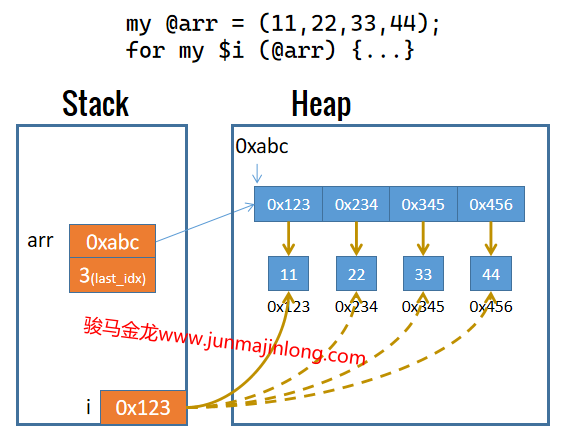## 遍历列表和数组，默认变量\$_

``````my @arr = qw(a b c d e f);

# while
my \$i = 0;
while(\$i <= \$#arr){
say "index: \$i, value: \$arr[\$i]";
\$i++;
}

# for
for(my \$i=0;\$i<=\$#arr;\$i++){
say "index: \$i, value: \$arr[\$i]";
}
``````

for或foreach的迭代数组或列表的方式如下。

``````my @arr = qw(a b c d e f);

# for
for my \$v (@arr) {
say "value: \$v";
}

# foreach
foreach my \$v (@arr) {
say "value: \$v";
}
``````

for或foreach迭代时，会从列表中逐个取元素，取一个元素，赋值给迭代控制变量`\$v`，然后执行一次循环体，再继续取下一个元素。

``````my @arr = (11, 22, 33, 44);
my \$v = 3333;
say \\$v;
say '---- before ----';
for my \$v (@arr) { say \\$v; }
say '---- after ----';
say \\$v;
say "\$v";
# 输出：
#   SCALAR(0x22f3d10)
#   ---- before ----
#   SCALAR(0x2277ab0)
#   SCALAR(0x2277a98)
#   SCALAR(0x2277b40)
#   SCALAR(0x2277b58)
#   ---- after ----
#   SCALAR(0x22f3d10)
#   3333
````````````my @arr = (11, 22, 33, 44);
for my \$v (@arr) {
\$v++;
}

say "@arr";  # 输出：12 23 34 45
``````

``````my @arr = (11,22,33,44,55,66);
for my \$i (@arr){
shift @arr;
say \$i;  # 11 33 55
}
``````

### 默认标量变量\$_

for和foreach迭代时需指定控制变量，例如`for \$i (...)`

``````for(11,22,33){ say \$_; }
for \$_ (11,22,33) { say \$_; }
``````

Perl的很多操作都允许省略操作目标，此时将使用默认变量`\$_`作为这些操作的操作目标。以后在使用Perl的过程中，自然会发现这一点。

``````chomp;
chomp \$_;
``````

print/say也可以省略参数，这时表示输出`\$_`的值。下面等价：

``````print;
print \$_;
``````

### 小心使用each

Perl提供了一个名为`each`的函数，它可用于迭代数组和hash。在列表上下文，each会返回数组的`(index, value)`或hash的`(key, value)`，在标量上下文，each会返回当前所处的indexkey

``````my @arr = (11,22,33,44);

# 列表上下文，each返回(index, value)
while(my (\$idx, \$v) = each @arr) {
say "index: \$idx, value: \$v";
}
``````

``````my @arr = (11,22,33,44);
for my \$v (@arr){
# 标量上下文，each返回index
say "index: ".(each @arr).", value: \$v";
}
``````

each取完最后一个元素后返回空列表或undef，其好处是可以直接在while循环中表示布尔假，使得取完所有元素后终止while循环。但如果不是each取完最后一个元素触发while循环的终止，那么任何使用each的地方都将出现不符合期待的结果。

``````my @arr = (11,22,33,44);
while(my (\$idx, \$v) = each @arr) {
say "index: \$idx, value: \$v";
last if \$idx == 2;
}
while(my (\$idx, \$v) = each @arr) {
say "index: \$idx, value: \$v";
}
``````

``````index: 0, value: 11
index: 1, value: 22
index: 2, value: 33
index: 3, value: 44
``````

``````my @arr = (11,22,33,44);
for my \$v (@arr){
say "index: ".(each @arr).", value: \$v";
}
for my \$v (@arr){
say "index: ".(each @arr).", value: \$v";
}
``````

``````index: 0, value: 11
index: 1, value: 22
index: 2, value: 33
index: 3, value: 44
index: , value: 11
index: 0, value: 22
index: 1, value: 33
index: 2, value: 44
``````